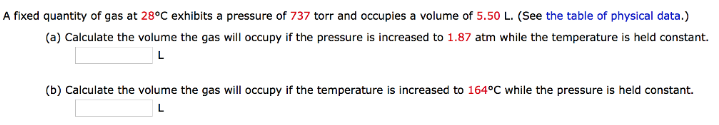# Problem: A fixed quantity of gas at 28°C exhibits a pressure of 737 torr and occupies a volume of 5.50 L. (See the table of physical data.) (a) Calculate the volume the gas will occupy if the pressure is increased to 1.87 atm while the temperature is held constant. (b) Calculate the volume the gas will occupy if the temperature is increased to 164°C while the pressure is held constant.

###### FREE Expert Solution
84% (313 ratings)###### Problem Details

A fixed quantity of gas at 28°C exhibits a pressure of 737 torr and occupies a volume of 5.50 L. (See the table of physical data.)

(a) Calculate the volume the gas will occupy if the pressure is increased to 1.87 atm while the temperature is held constant.

(b) Calculate the volume the gas will occupy if the temperature is increased to 164°C while the pressure is held constant.